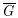Problems

# Good Graphs

Alex defined good graphs:

• Single vertex is a good graph.
• If two good graph have no common vertex then their union is a good graph.
• If G is a good graph then(complement of G) is a good graph.

Try to solve the problem of finding maximal weighted clique in a good graph.

Input

The first line of contains the integer N (1N500) - number of vertices in the good graphG.

The next N lines contain adjacency matrix of G.

Each of last N lines contains the integer wi (1wi1000) - the weight of ith vertex.

Output

In the single line of the output file print the maximal weight of clique of graph G.

Time limit 1 second
Memory limit 64 MiB
Input example #1
4
0000
0011
0101
0110
100
1
2
3

Output example #1
100